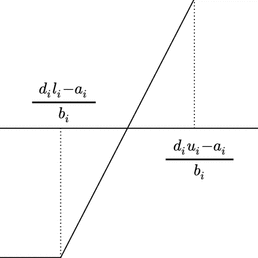A Newton’s method for the continuous quadratic knapsack problem

Roberto Cominetti, Walter F. Mascarenhas, Paulo J. S. Silva. Mathematical Programming Computations, 2014.

# Abstract

We introduce a new efficient method to solve the continuous quadratic knapsack problem. This is a highly structured quadratic program that appears in different contexts. The method converges after O(n) iterations with overall arithmetic complexity O(n2). Numerical experiments show that in practice the method converges in a small number of iterations with overall linear complexity, and is faster than the state-of-the-art algorithms based on median finding, variable fixing, and secant techniques.